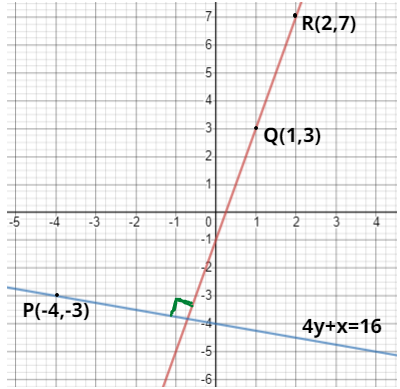Courses
Courses for Kids
Free study material
Free LIVE classes
MoreLIVE
Join Vedantu’s FREE Mastercalss

# ${\text{Find the equation of the line passing through the point }}\left( { - 4, - 3} \right){\text{ and perpendicular to the straight }} \\ {\text{line joining }}\left( {1,3} \right){\text{ and }}\left( {2,7} \right). \\$Verified${\text{Now, we have to find the equation of line passing through point }}P\left( { - 4, - 3} \right){\text{ and perpendicular}} \\ {\text{to the line joining the given points}}{\text{.}} \\ {\text{First we find slope of line formed after joining two points Q}}\left( {1,3} \right){\text{ and }}R\left( {2,7} \right){\text{.}} \\ {\text{Let slope of line joining points Q}}\left( {1,3} \right){\text{ and }}R\left( {2,7} \right){\text{ be }}{m_1}{\text{.}} \\ {\text{Them }}{m_1} = \dfrac{{{y_2} - {y_1}}}{{{x_2} - {x_1}}} = \dfrac{{7 - 3}}{{2 - 1}} = 4 \\ {\text{Let the slope of required line be }}{m_2} \\ {\text{As, it is give that the required line is perpendicular to the line joining points Q}}\left( {1,3} \right){\text{ and }}R\left( {2,7} \right). \\ {\text{So, }}{m_1}*{m_2} = - 1 \\ {\text{Putting value of }}{m_1}{\text{, we get }}{m_2} = \dfrac{{ - 1}}{4} \\ {\text{Now, finding the equation of line passing through point P and having slope }}{m_2}{\text{ using point slope form,}} \\ \left( {y - \left( { - 3} \right)} \right) = \dfrac{{ - 1}}{4}\left( {x - \left( { - 4} \right)} \right){\text{ }}\left( 1 \right) \\ {\text{Cross - multiplying equation 1 we get,}} \\ 4y + 12 = - x - 4 \\ {\text{Solving above equation we get }}4y + x + 16 = 0 \\ {\text{Hence, equation of line passing through point P and perpendicular to line joining points Q and R}} \\ {\text{is }}4y + x + 16 = 0 \\ {\text{NOTE: - Whenever you come up with a type of problem the first find the slope of lines}}{\text{. As for}} \\ {\text{perpendicular lines }}{m_1}*{m_2} = - 1{\text{ and for parallel line }}{m_1} = {m_2}{\text{. Then use point - slope form to }} \\ {\text{find equation of line}}{\text{.}} \\$